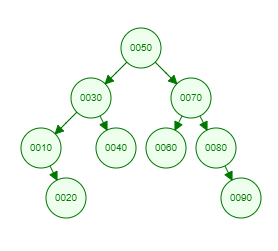Recent Tutorials and Articles
Printing Left View of Binary Tree in Java
Published on: 27th October 2018

This tutorial provides a Java program to print nodes of Binary Tree that will be visible from left view.

# Understanding Left View of a Binary Tree

Left View of a Binary Tree consists of nodes that are visible from Left side of a Binary Tree. This requires level order traversal and printing first node from left at each level.

Let's consider below Binary Tree -Here is the left view of above Binary tree -

``````50
30
10
20
``````

# Java Implementation of Left View of a Binary Tree

Here is Java code for Binary Tree containing methods for inserting data and printing left view of Binary Tree -

``````package com.aksain.data.structures;

import java.util.Queue;

public class BinaryTreeWithLeftView<T extends Comparable<T>> {
private Node root;

private class Node {
T data;
Node left;
Node right;

private Node(T data) {
this.data = data;
}
}

private Node doInsert(T data, Node node) {
if (node == null) {
return new Node(data);
}
int result = data.compareTo(node.data);
if (result < 0) {
node.left = doInsert(data, node.left);
} else if (result > 0) {
node.right = doInsert(data, node.right);
}

return node;
}

public void insert(T data) {
root = doInsert(data, root);
}

private void doPrintLeftView(Node root) {
final Queue<Node> queue = new LinkedList<>();

while(!queue.isEmpty()) {
int noOfElementsAtThisLevel = queue.size();
for(int index = 0; index < noOfElementsAtThisLevel; index++) {
Node tmp = queue.poll();
if(index == 0) {
System.out.print(tmp.data + " ");
}

if(tmp.left != null) {
}
if(tmp.right != null) {
}
}
System.out.println();
}
}

public void printLeftView() {
doPrintLeftView(root);
}

public static void main(String[] args) {
final BinaryTreeWithLeftView<Integer> binaryTree = new BinaryTreeWithLeftView<>();
binaryTree.insert(50);
binaryTree.insert(30);
binaryTree.insert(10);
binaryTree.insert(20);
binaryTree.insert(40);
binaryTree.insert(70);
binaryTree.insert(60);
binaryTree.insert(80);
binaryTree.insert(90);

binaryTree.printLeftView();
}
}
``````

Here is the output of above code -

``````50
30
10
20
``````

Thank you for reading through the tutorial. In case of any feedback/questions/concerns, you can communicate same to us through your comments and we shall get back to you as soon as possible.

Published on: 27th October 2018